•Gurobi Staff

First, let me answer your specific questions,

...The problem was solved successfully this time, but the calculation speed became very slow after the results were used for the next input of the iterative algorithm.  Why does the second calculation in the iterative algorithm become very slow after successfully solving the first calculation, and the gap values are difficult to appear？

One thing that stands out in the log of the 'next iteration' provided are the $$\texttt{max constraint violation}$$ and $$\texttt{max general constraint violation}$$ warnings. Warnings like this mean that the solver encountered some numerical issues during the solving process. There are various reasons for this. More on how to fix this below.  However, this means the solution is not feasible.  According to the log, the maximum violation is about 20 --- which is quite large.  If you want to see which constraint is associated with this violation, you can run model.printQuality().

Providing a feasible starting point typically helps when you resolve a model.  If your iterative algorithm is intended to provide feasible starting points for the next solve, it should start with an incumbent solution and gap value.  As you noticed, your log file is showing that the 'next iteration' model does not have a feasible starting point.  This is because either (a) the previous iteration's solution is naturally infeasible for the 'next iteration' model (because of added constraints) or (b) the previous iteration's solve also terminated with $$\texttt{max constraint violation}$$ warnings.  Did you see violation warnings in the previous iteration's log?

Why do I set MIPGap = 0.0003 and the solver calculation does not stop when the gap reaches 0.03%? But the first time I set MIPGap = 0.00005, solver stopped running after the gap reached 0.05%.

The log gap value is rounded to two decimal places.  If I recalculate the last row by hand, I get $$\left(12587.9063 - 12584.0635\right) / 12584.0635 = 0.0305\%$$.  This value has not met the 0.03% tolerance.

Next, here are a few tips to improve the solver performance:

• Numerical Stability:
Your biggest performance hurdle appears to be numerical stability.  This affects both the solution time and the solution quality. The best thing you can do is reformulate the model to reduce the range of matrix coefficients. For more information on how to make improvements, see Guidelines for Numerical Issues.  As a workaround, you can try different combinations of the following parameters: NumericFocus = 1, 2, or 3; ScaleFlag = 2; Aggregate = 0; and PreMIQCPForm = 0, 1, or 2.
• After you have addressed the numerical stability issues:
Once you have the numerical stability taken care of, you can start looking at other options to reduce solution time.  Here is where looking at different levels of MIPFocus or using Gurobi's automatic Parameter Tuning Tool can help.

•Hi,

Thank you very much for your detailed answer. Through your explanation, I understand why I set MIPGap failed. Through your suggestion, I used model.printQuality() to print out more detailed information, but I still don't know the problem of the warning of Max constraint violation and Max general constraint violation.

• Could you help me point out which constraint is wrong?

Strangely, I don't know what has been modified. It's difficult to reduce gap when it reaches about 0.6%. In order to make model.printQuality() run successfully, I set MIPGap to 0.006 instead of the original 0.0005.

Here are the details printed by model.printQuality().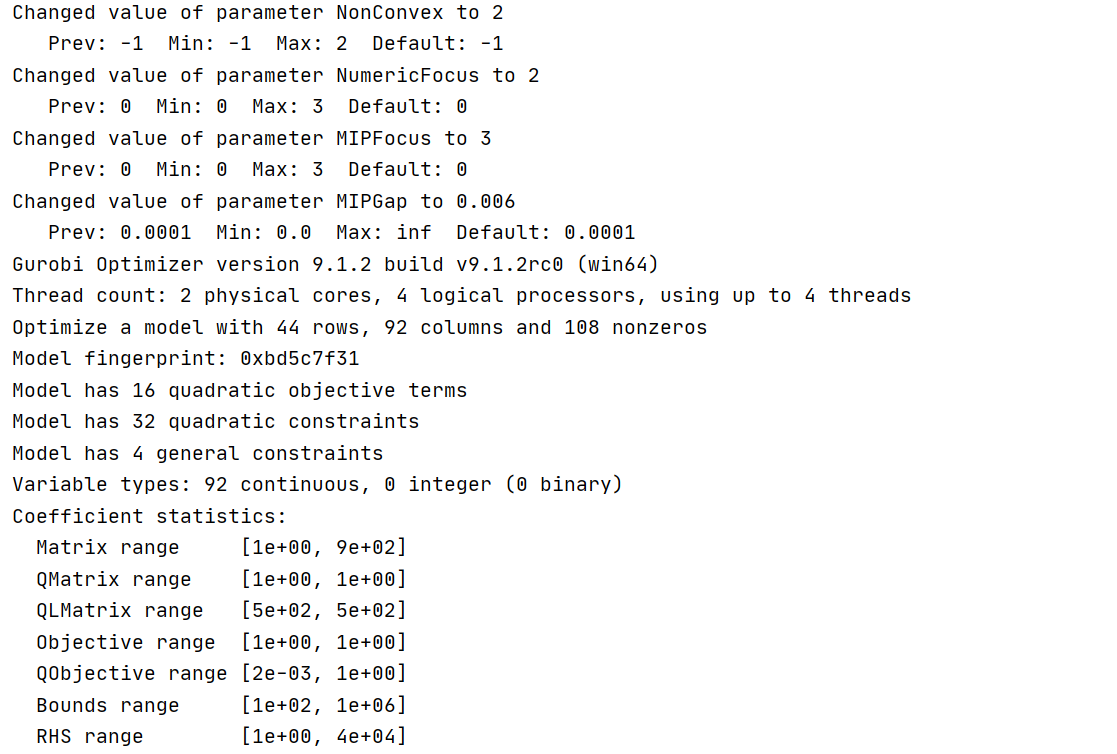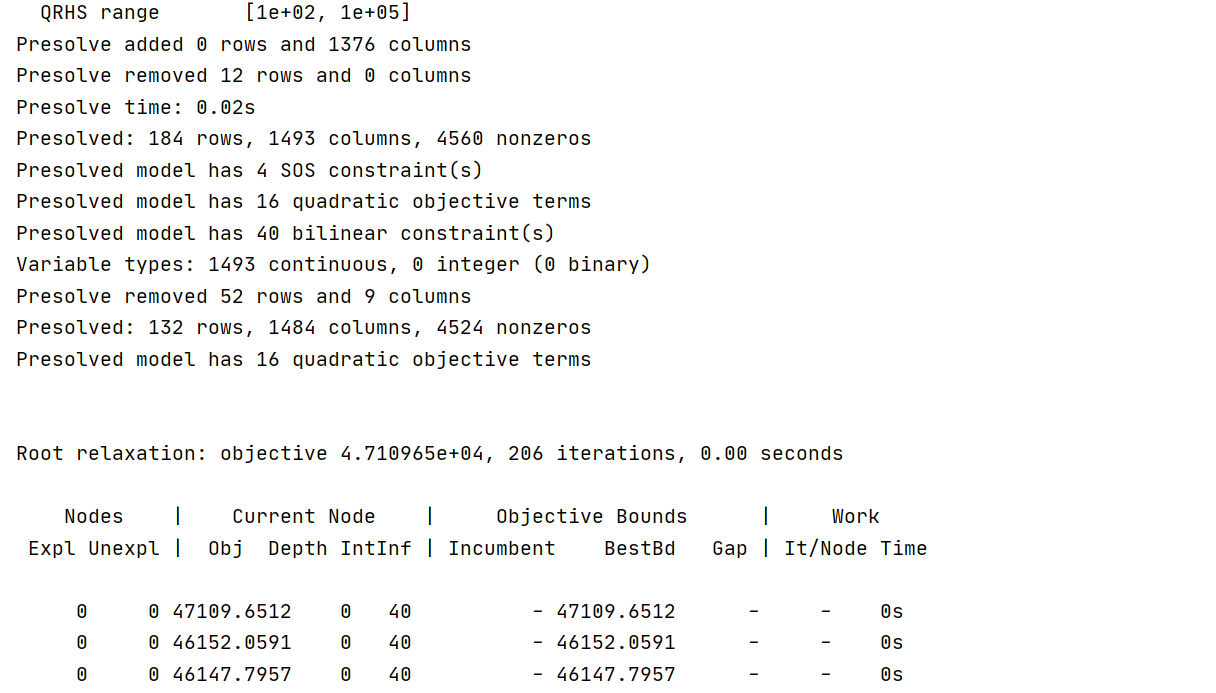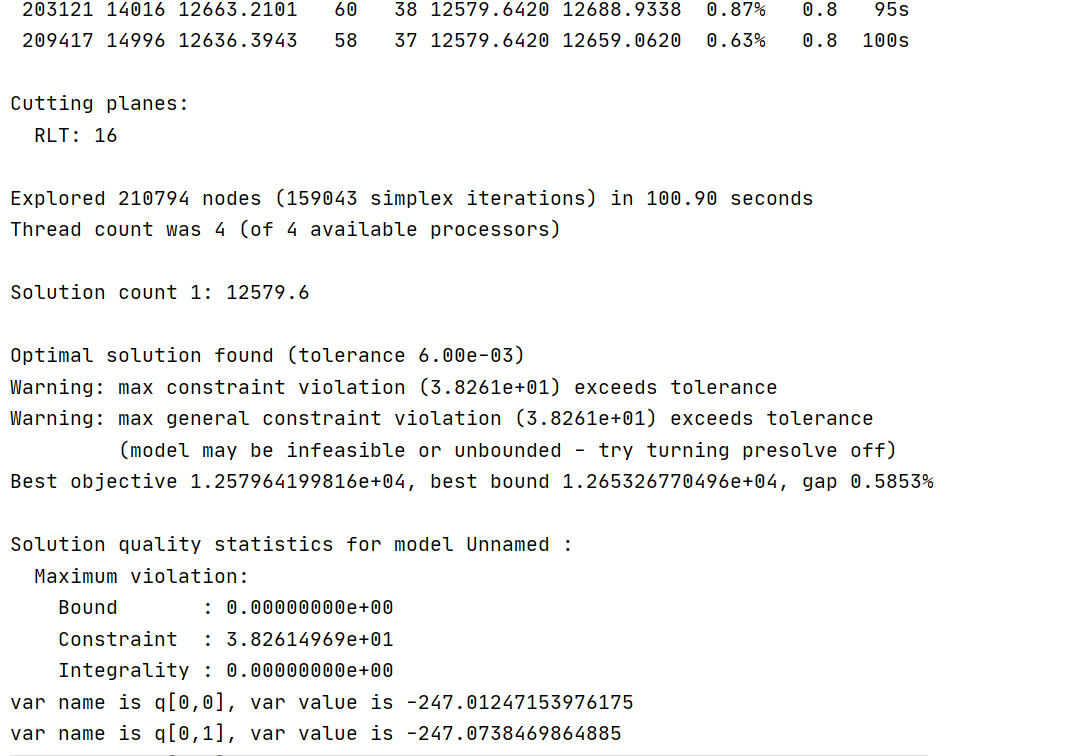About the Numerical Stability，I tried to modify the settings of parameters such as ScaleFlag and  Aggregate, but did not improve the solution time, and the second iteration could not be completed smoothly.

Because I am not familiar with gurobi, I use a lot of constraints when expressing the objective function. Will the number of constraints also affect the solving speed of solver? Can the objective function be expressed more optimally?

• Could you help me see if there is any way to shorten the solution time and make the iterative algorithm run successfully?

Finally, I'm sorry for the trouble I caused you. Thank you again for your careful answer. Here is my code.

def gurobi_demo(qc):    model = grb.Model('')    s = np.array([[-250, -250, 0], [-250, 250, 0], [250, -250, 0], [250, 250, 0]])    q = model.addVars(4, 3, lb=-300, vtype=GRB.CONTINUOUS, name='q')    z = model.addVars(4, 4, vtype=GRB.CONTINUOUS, name='z')    zs = model.addVars(4, 4, vtype=GRB.CONTINUOUS, name='zs')    term4 = model.addVars(4, vtype=GRB.CONTINUOUS, name='term4')    term3 = model.addVars(4, vtype=GRB.CONTINUOUS, name='term3')    term2 = model.addVars(4, 4, lb=-100, vtype=GRB.CONTINUOUS, name='term2')    l = model.addVars(4, 4, vtype=GRB.CONTINUOUS, name='l')    lv = model.addVars(4, lb=-1000000, vtype=GRB.CONTINUOUS, name='lv')    dc = np.array([[0, 0, 0, 0], [0, 0, 0, 0], [0, 0, 0, 0], [0, 0, 0, 0]])    for k in range(4):        for j in range(4):            dc[k, j] = np.linalg.norm(qc[j] - s[k]) / 10    term1 = {}    for k in range(K):        term1[k] = 1        for o in range(K):            term1[k] += 1 / (np.linalg.norm(qc[o] - s[k]) ** 2)    model.update()    sum = LinExpr()    for k in range(K):        sum += math.log(term1[k])        for j in range(K):            model.addConstr(term2[k, j] == -1 * 1.44 * 10000 * (l[k, j] * l[k, j] - np.linalg.norm(qc[j] - s[k]) ** 2) / dc[k, j] ** 4)            sum += term2[k, j] / term1[k]                for j in range(K):            model.addConstr(z[k, j] * zs[k, j] == 100)            model.addConstr(z[k, j] <= np.linalg.norm(qc[j] - s[k]) ** 2 + 2 * (                grb.quicksum((qc[j][i] - s[k][i]) * (q[j, i] - qc[j][i]) for i in range(3))))        model.addConstr(term3[k] * 100 == grb.quicksum(zs[k, j] for j in range(K) if j != k))        model.addConstr(term4[k] == term3[k] + 1)        # lv = log2(term3)        model.addGenConstrLogA(term4[k], lv[k], 2, "log2")        sum += -1 * lv[k]    model.setObjective(sum, sense=GRB.MAXIMIZE)    # -------------------------------------------------------------------    for k in range(K):        model.addConstr(q[k, 2] == 100)        model.addConstr(q[k, 0] <= 300)        model.addConstr(q[k, 0] >= -300)        model.addConstr(q[k, 1] <= 300)        model.addConstr(q[k, 1] >= -300)    # ---------------------------------------    # np.linalg.norm(q[j] - s[k])    for k in range(K):        for j in range(K):            rhs = QuadExpr()            for i in range(3):                rhs += q[j, i] * q[j, i] - 2 * s[k][i] * q[j, i]            model.addConstr(l[k, j] * l[k, j] == rhs + s[k] ** 2 + s[k] ** 2 + s[k] ** 2)    model.setParam('NonConvex', 2)    model.setParam('NumericFocus', 2)    model.setParam('MIPFocus', 3)    # model.setParam('ScaleFlag', 2)    # model.setParam('Aggregate', 0)    model.setParam('MIPGap', 0.006)    model.optimize()    model.printQuality()    for v in model.getVars():        print('var name is {}, var value is {}'.format(v.VarName, v.X))    return [[q[i, j].X for j in range(3)] for i in range(4)]
def main():    qc = [[[-200, -200, 100], [-200, 200, 100], [200, -200, 100], [200, 200, 100]]]    while True:        qc.append(gurobi_demo(qc[-1]))        print(qc)
•Gurobi Staff
Could you help me point out which constraint is wrong?

I recommend looking at the Slack attribute of all of your constraints (QCSlack for quadratic constraints).  The slack value is the difference between the right-hand side and the left-hand side of the constraint.  In theory, the slack values and distance to variable limits should be nonnegative. However, Gurobi will consider any value within 1e-6 of the bound or RHS as feasible by default. This is controlled by the FeasibilityTol parameter.   The most largely negative slacks are your most violated constraints.

About the Numerical Stability，I tried to modify the settings of parameters such as ScaleFlag and  Aggregate, but did not improve the solution time, and the second iteration could not be completed smoothly.

Your first goal should be to use improve feasibility.  Once you have a model that solves without any $$\texttt{max violation}$$ warnings, then you can start to address the speed.  So, when changing these parameters, check model.printQuality().  If the violations are decreasing, you are going in the right direction.

I also recommend including combinations of NumericFocus = 1, 2, or 3 and PreMIQCPForm = 0, 1, or 2 alongside your experiments with ScaleFlag = 2 and Aggregate = 0.  Remember, these parameters are only a workaround; the most reliable fix is reformulating the model.

We are often asked how to reformulate a model to improve numerical stability.  As a result, we have put together a reference manual to help: Guidelines for Numerical Issues. In particular, the Tolerance and user-scaling and Advanced User-scaling sections may prove useful.

•Hi,

Thank you for your advice. Let me know that I should first solve the problem of max violation warnings and then solve the performance problem of solver.

And then, There are two types of  warning problems I face, max constraint violation warning and max general constraint violation. I first solved the warning of linear constr. I queried the slack of linear constr. I named linear constrsC0, C1...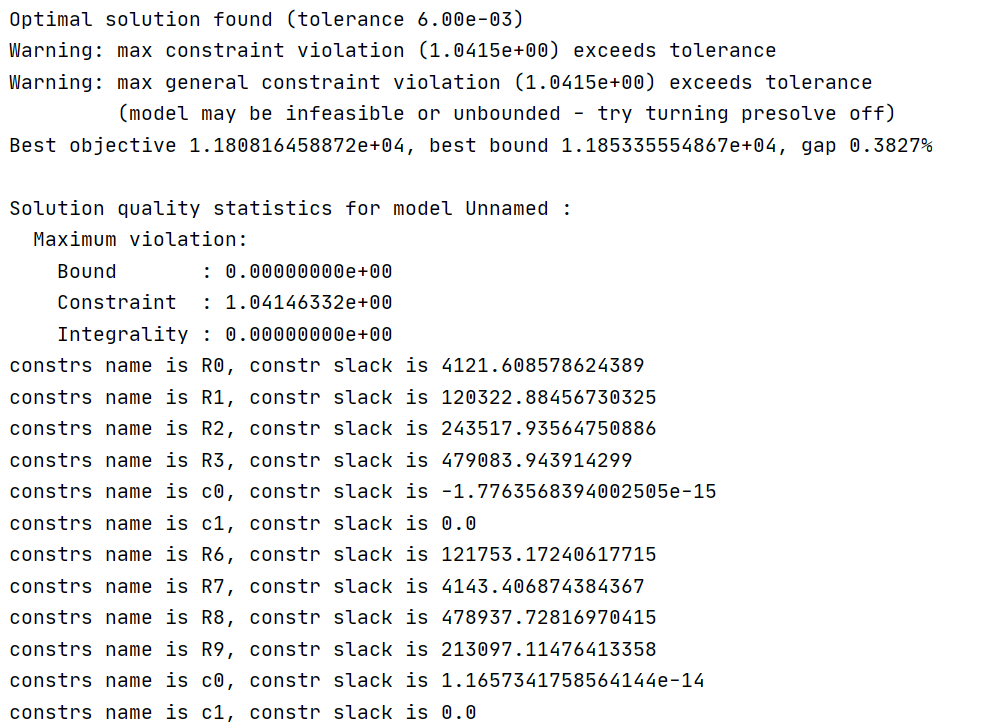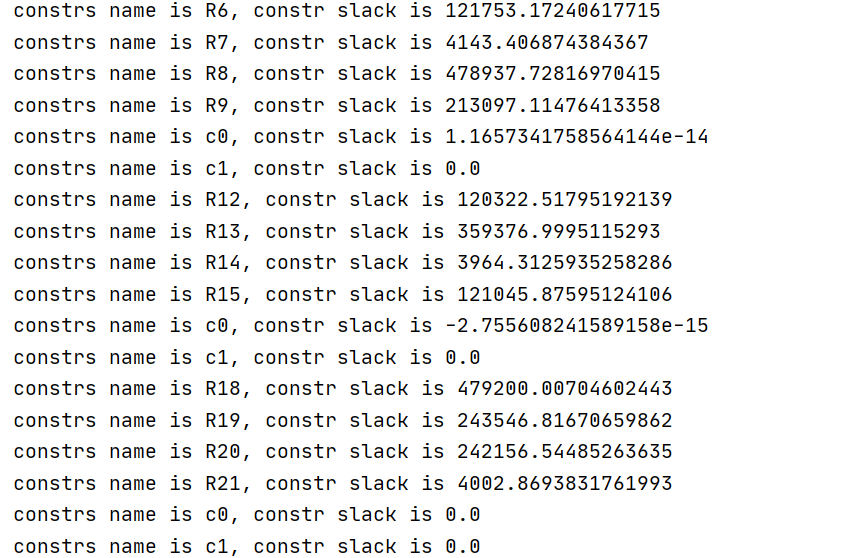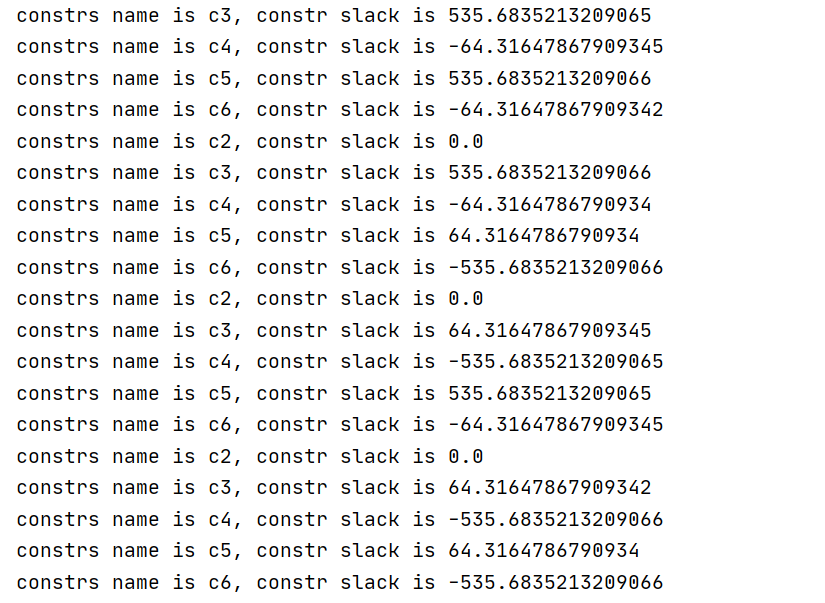• I did not set R0,R1.... what constr do these R0,R1... refer to?
• Since my  FeasibilityTol parameter is default and my C0 is negative, is there the violated problem in my C0?

The following is constr C0.

model.addConstr(term3[k] == grb.quicksum(zs[k, j] for j in range(K) if j != k), "c0") model.addConstr(term4[k] == term3[k] + 1, "c1")

Because the slack value is the difference between the right-hand side and the left-hand side of the constraint. I changed C0 to the following style to reduce slack.

model.addConstr(term3[k] * 1000000 == grb.quicksum(zs[k, j] for j in range(K) if j != k), "c0")model.addConstr(term4[k] == term3[k] * 10000 + 1, "c1")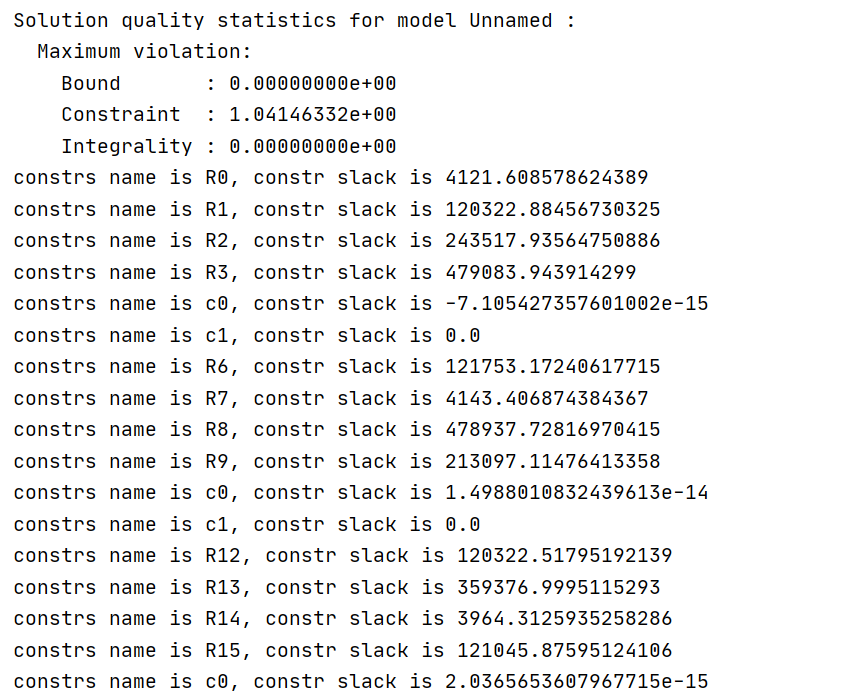• Has my operation improved the warning problem?
• But the warning still exists. How can C0 improve to solve the problem of warning?

Best regards,

Yanbo

•Gurobi Staff

The conversation is continued in Some problems about parameter selection and Max constraint violation exceeded tolerance

•Hi Alison，

Okay! Thank you very much for your help!

Best regards,

Yanbo